Stability Analysis of Slopes

# Stability Analysis of Slopes - Notes | Study Civil Engineering SSC JE (Technical) - Civil Engineering (CE)

 1 Crore+ students have signed up on EduRev. Have you?

Chapter 11
Stability Analysis of Slopes
:
Factor of safety:
Factor of safety of a slope is defined as the ratio of average shear strength (Ƭf) of a soil to the average shear stress (Ƭd) developed along the potential failure surface.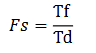Fs = Factor of safety
Ƭf= average shear strength of the soil
Ƭd= average shear stress developed along the potential surface
Shear Strength:
Shear strength of a soil is given by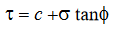Where, c = cohesion
Φ= angle of internal friction
σ= Normal stress on the potential failure surface
Similarly, the mobilized shear strength is given by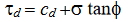Cd and Φd are the cohesion and angle of internal friction that develop along thepotential failure surface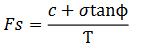Fs w.r.t cohesion is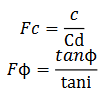SLOPES:
1. Infinite Slope
2. Finite Slope

1. Infinite Slope:- If the slopes represents the boundary surface of infinite soil man & the properties of the soil at similar depth below the ground, it is termed as infinite slope.
-Failure take place due to ‘sliding’ 
-Failure surface is parallel to the ground plane or slope

2. Finite slope:-
-Slopes are of finite extent bounded by top and bottom surfaces 
-Failure take place due to rotation. 
-Failure plane is either circular spiral

STABILITY ANALYSIS OF INFINITE SLOPES:
(i) Cohesionless dry soil/dry sand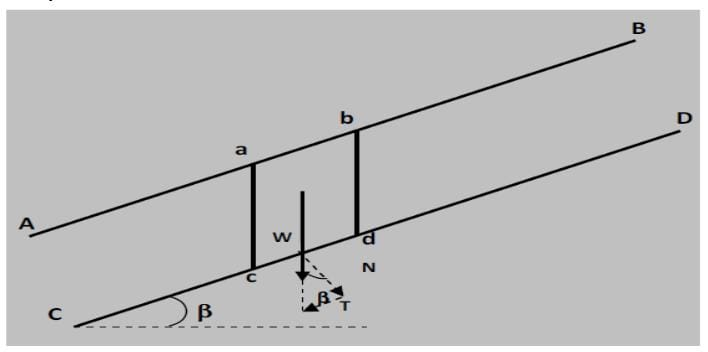The factor of safety against sliding failure is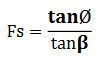Under the limiting equilibrium Fs =1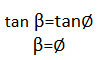“The maximum inclination of an infinite slope in cohesion less soil for stability is equal to the angle of internal friction of the soil”.The limiting angle of inclination for stability of an infinite slope in cohesionless soils as shown below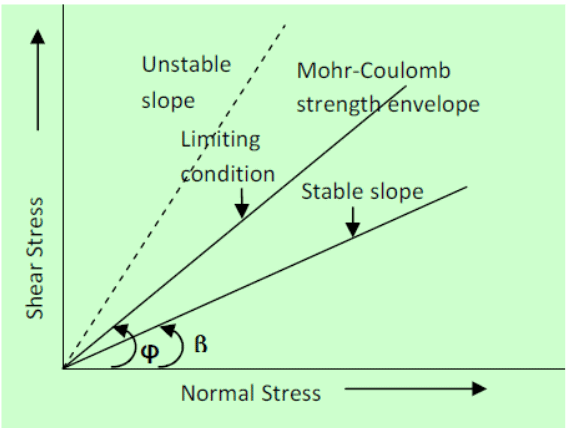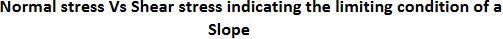(ii) Seepage taking place and water table is parallel to the slope in Cohesionless soil

- Water table at a height h above the the failure plane:
Fs=(1-γw.h/γ.z).tanΦ/tanβ
(iii) If water table is at ground level : i.e. h=z

Fs=γ'tanΦ/γtanβ

(v) Infinite Slope of Purely Cohesive Soil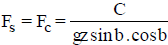Here H = z = depth of slice/cut.
At critical stage Fc =1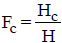where Sh = Stability Number.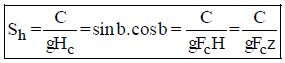(vi) C-f soil in Infinite Slope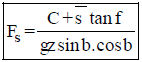STABILITY ANALYSIS OF FINITE SLOPES
(i) Fellinious method (For purely Cohesive Soil)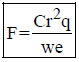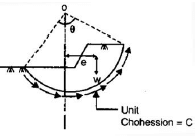where F = Factor of safety
r = Radius of rupture curve
l = length of rupture curve

(b)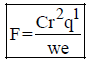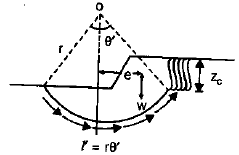Factor = Factor of safety it tension cracks has developed.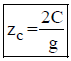(ii) Swedish Circle Method

F=(c'L+tanΦ∑W cosα)/∑wsinα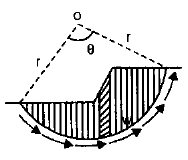where, F = Factor of safety

(iii) Friction Circle Method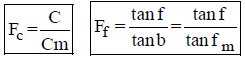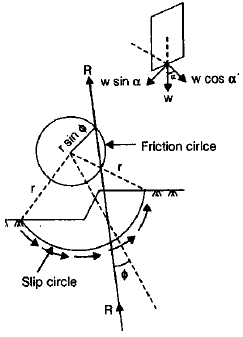(iv) Taylor’s Stability Method (c -f soil)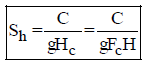In case of submerged slope g' should be used instead of g and if slope is saturated by capilary  flow then gsat should be used instead of g.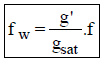where fw = Weight friction angle.

The document Stability Analysis of Slopes - Notes | Study Civil Engineering SSC JE (Technical) - Civil Engineering (CE) is a part of the Civil Engineering (CE) Course Civil Engineering SSC JE (Technical).
All you need of Civil Engineering (CE) at this link: Civil Engineering (CE)

## Civil Engineering SSC JE (Technical)

2 videos|122 docs|50 tests
 Use Code STAYHOME200 and get INR 200 additional OFF

## Civil Engineering SSC JE (Technical)

2 videos|122 docs|50 tests

Track your progress, build streaks, highlight & save important lessons and more!

,

,

,

,

,

,

,

,

,

,

,

,

,

,

,

,

,

,

,

,

,

;